# ISEE Lower Level Math : How to find the perimeter of a trapezoid

## Example Questions

### Example Question #1 : How To Find The Perimeter Of A Trapezoid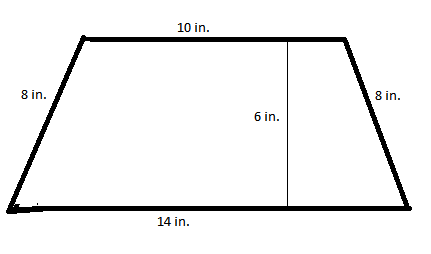Figure not drawn to scale

Find the perimeter of the trapezoid.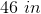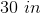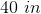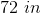Explanation:

In order to find the perimeter of the trapezoid, you must add up the lengths of all the sides of the trapezoid.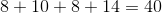Because the lengths of the trapezoid are in inches, the answer is 40 in.

### Example Question #2 : How To Find The Perimeter Of A Trapezoid

What would you call a polygon with four sides and four angles, all of different measurements?

Rhombus

None of these

Rectangle

Square

Explanation:

A quadrilateral has four sides and four angles, but no restrictions on how the lengths or measures are related. Thus, quadrilateral is the right answer.

In squares and rectangles, all four angles measure 90 degrees; thus the angles are all of equal measurements, making square and rectangle incorrect answer choices. A rhombus has two sets of angles of equal measurements, and would also be incorrect.

### Example Question #1227 : Isee Lower Level (Grades 5 6) Mathematics Achievement

Mrs. Green's garden is shaped like a trapezoid.  She wants to build a fence on the perimeter of her garden.  The sides of her garden measure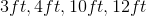. How long will the fence need to be?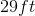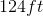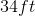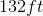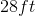Explanation:

To calculate the perimeter of a trapezoid, find the sum of the four sides.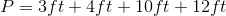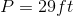### Example Question #3 : How To Find The Perimeter Of A Trapezoid

The perimeter of a trapezoid is two times the base length plus two times the side lengths. True or False?

False

True

False

Explanation:

Since the two base lengths area not the same length, you must add them separately and not just multiple one of the by two.

### Example Question #5 : How To Find The Perimeter Of A TrapezoidFigure not drawn to scale

Find the perimeter of the trapezoid.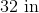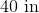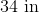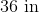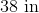Because the lengths of the trapezoid are in inches, the answer is